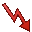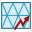# AV Card

This card defines an impressed current source similar to the AI card but that makes electrical contact with a conducting surface.

On the Source/Load tab, in the Ideal sources group, click theImpressed current icon. From the ドロップダウンリスト, click theImpressed current connected to mesh vertex (AV) icon.

The current varies linearly between the value at the start point and that at the end point. At the connection point special singular functions are used for the surface current density on the triangles to allow continuous current flow.

## Parameters:

New source
A new excitation is defined which replaces all previously defined excitations.
A new excitation is defined which is added to the previously defined excitations.
Specify end point coordinates
The coordinates of the end point r2 are specified with the X, Y, Z coordinate fields. This point must coincide with a corner point of one or more triangles.
Connect to closest triangle vertex
The coordinates of the end point r2 are not known. In this case the X, Y, Z coordinate fields of the end point are not used. Feko searches through all the metallic triangles for the corner point that is closest to the start point r1 of the current element. This is then the end point r2.
Connect to closest segment vertex
The coordinates of the end point r2 are not known. In this case the X, Y, Z coordinate fields of the end point are not used. Feko searches through all the metallic segments for the vertex that is closest to the start point r1 of the current element. This is then the end point r2.
Amplitude (A)
Current amplitude (in A) at the start, r1, and end, r2, points.
Phase (deg.)
Phase of the current at the start point in degrees.
X, Y, Z coordinate
Coordinates of the start and end points in m. (Note that all the coordinate values are optionally scaled by the SF card.)